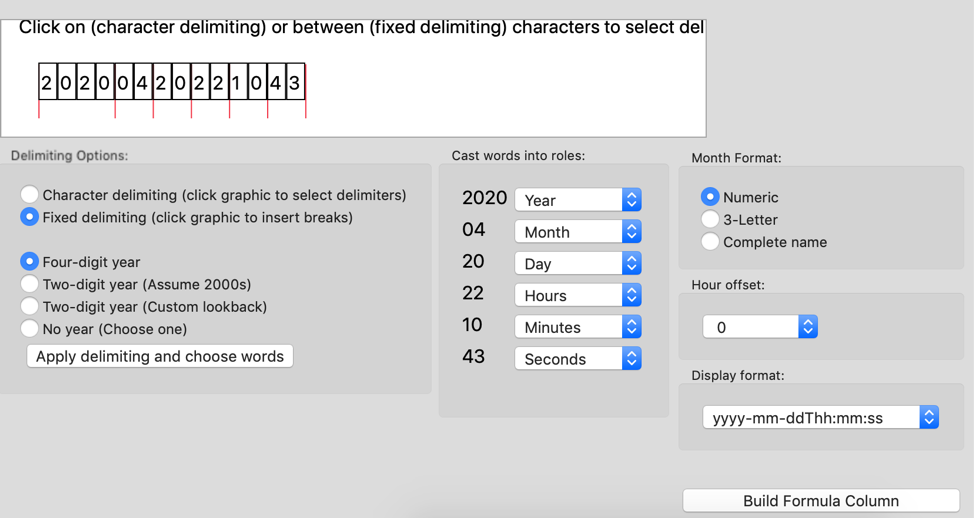Choose Language Hide Translation Bar
Highlighted

## Coverting from numeric strings

Hi

I would like covert numeric values into a date. I tried many of sample scripts here but it didn't work.

I have 14 digits in a column that should be transformed such as 20200420221043. I want yyyy-mm-ddThh:mm:ss type. Therefore, it seems like 2020-04-20T22:10:43.

Any ideas?

1 ACCEPTED SOLUTION

Accepted Solutions
Highlighted

## Re: Coverting from numeric strings

Just in case you want a column that is a numeric date that is formatted, here is a script to do that. It creates a 1 column table. Extending @txnelson's solution, the string column is called myS. The script creates a date column, myD. The wait(2) is added for dramatic effect.

Just a 2nd approach.

``````Names Default to Here(1);

dt = New Table( "Test",
New Column( "myS",
Character,
"Nominal",
Set Values( {"20200420221053", "20200419112025", "20191225055920"} )
)
);

dt << New Column("myD", Numeric, Continuous);

Column(dt,"myD") << set each value(Informat(substr(:myS,1,8), "YYYYMMDD") + num(Substr(:myS,9,2))*60*60
+ num(substr(:myS,11,2))*60 + num(substr(:myS,13,2)) );

wait(2);

:myD << Format("yyyy-mm-ddThh:mm:ss", 19, 0);

``````
8 REPLIES 8
Highlighted

## Re: Coverting from numeric strings

Is this what you are looking for?

``````myS = "20200420221043";

datex = Informat(
Substr( myS, 1, 4 ) || "/" || Substr( myS, 5, 2 ) || "/" || Substr( myS, 7, 2 ) || " " ||
Substr( myS, 9, 2 ) || ":" || Substr( myS, 11, 2 ) || ":" || Substr( myS, 13, 2 ),
"y/m/d h:m:s"
);

Show( Format( datex, "yyyy-mm-ddThh:mm:ss" ) );``````
`Format(datex, "yyyy-mm-ddThh:mm:ss") = "2020-04-20T22:10:43";`
Jim
Highlighted

## Re: Coverting from numeric strings

Just in case you want a column that is a numeric date that is formatted, here is a script to do that. It creates a 1 column table. Extending @txnelson's solution, the string column is called myS. The script creates a date column, myD. The wait(2) is added for dramatic effect.

Just a 2nd approach.

``````Names Default to Here(1);

dt = New Table( "Test",
New Column( "myS",
Character,
"Nominal",
Set Values( {"20200420221053", "20200419112025", "20191225055920"} )
)
);

dt << New Column("myD", Numeric, Continuous);

Column(dt,"myD") << set each value(Informat(substr(:myS,1,8), "YYYYMMDD") + num(Substr(:myS,9,2))*60*60
+ num(substr(:myS,11,2))*60 + num(substr(:myS,13,2)) );

wait(2);

:myD << Format("yyyy-mm-ddThh:mm:ss", 19, 0);

``````
Highlighted

## Re: Coverting from numeric strings

I tried this script and it worked well.

Especially, 'wait()' makes me fun.

Highlighted

## Re: Coverting from numeric strings

Great reference as well.

Highlighted

## Re: Coverting from numeric strings

Hi,

There is an addin for exactly this type of problem. Find the addin and a description of how to use it, here: Data table tools, part 1: Custom Date Formula Writer

After opening the addin file to install it, it will be located in the "Addins" section of JMP's main menu. Select: Addins > Data Table Tools > Special Formula Columns > Custom Date Formula Writer.

In the main window of the Formula Writer, select your data table and column of source data--be sure to set your data to charater & nominal types.

Then, fill out the rest of the dialog as below, being sure to place tickmarks at the beginning and end of all fields of interest. (Clicking toggles the tickmarks on and off.)

Click the "Build Formula Column" and you're done. (Example of the auto-written formula far below.) Note that there are zeros and empty braces in various places, representing options which were not used in the present formula. This is as intended.

Cheers,``````Try(
{};
YearChoice = 1;
{};
{};
Date MDY(
Num( Substr( :DateStr, 5, 2 ) ),
Num( Substr( :DateStr, 7, 2 ) ),
Num( Substr( :DateStr, 1, 4 ) ) + 0
) + Num( Substr( :DateStr, 13, 2 ) ) + Num( Substr( :DateStr, 11, 2 ) ) * 60 + (
Num( Substr( :DateStr, 9, 2 ) ) + 0) * 3600 + If( Num( Substr( :DateStr, 9, 2 ) ) < 12,
0,
0
);
)``````

Highlightedstan_koprowskiCommunity Manager

## Re: Coverting from numeric strings

Hi @KrissKdash,

Also, be sure watch the videoThe Doctor Cures  Your Date and Time Import Problems.

In this segment which recently aired @brady_brady demonstrates step by step how to solve these types of date problems.

cheers,

Stan

Highlighted

Great reference.
Highlighted

## Re: Coverting from numeric strings

I need to bookmark this. Thanks.
Article Labels

There are no labels assigned to this post.